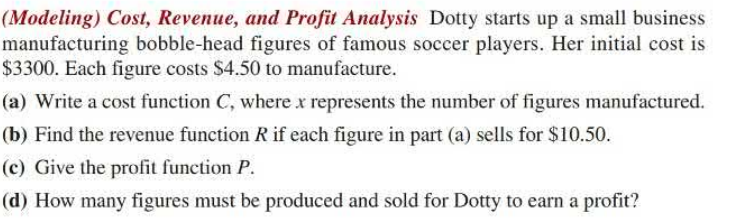### Still have math questions?

Algebra
Question(Modeling) Cost, Revenue, and Profit Analysis Dotty starts up a small business manufacturing bobble-head figures of famous soccer players. Her initial cost is $$\ 3300$$ . Each figure costs $$\ 4.50$$ to manufacture.

(a) Write a cost function $$C$$ , where $$x$$ represents the number of figures manufactured.

(b) Find the revenue function $$R$$ if each figure in part (a) sells for $$\ 10.50$$ .

(c) Give the profit function $$P$$ .

(d) How many figures must be produced and sold for Dotty to earn a profit?

$$\left. \begin{array} { l l } { \text { [2.4] 22. } \left. \begin{array} { l l } { \text { (a) } C ( x ) = 3300 + 4.50 x } & { \text { (b) } R ( x ) = 10.50 x } \\ { \text { (c) } R ( x ) - C ( x ) = 6.00 x - 3300 } & { \text { (d) } 551 \text { figures } } \end{array} \right. } \end{array} \right.$$